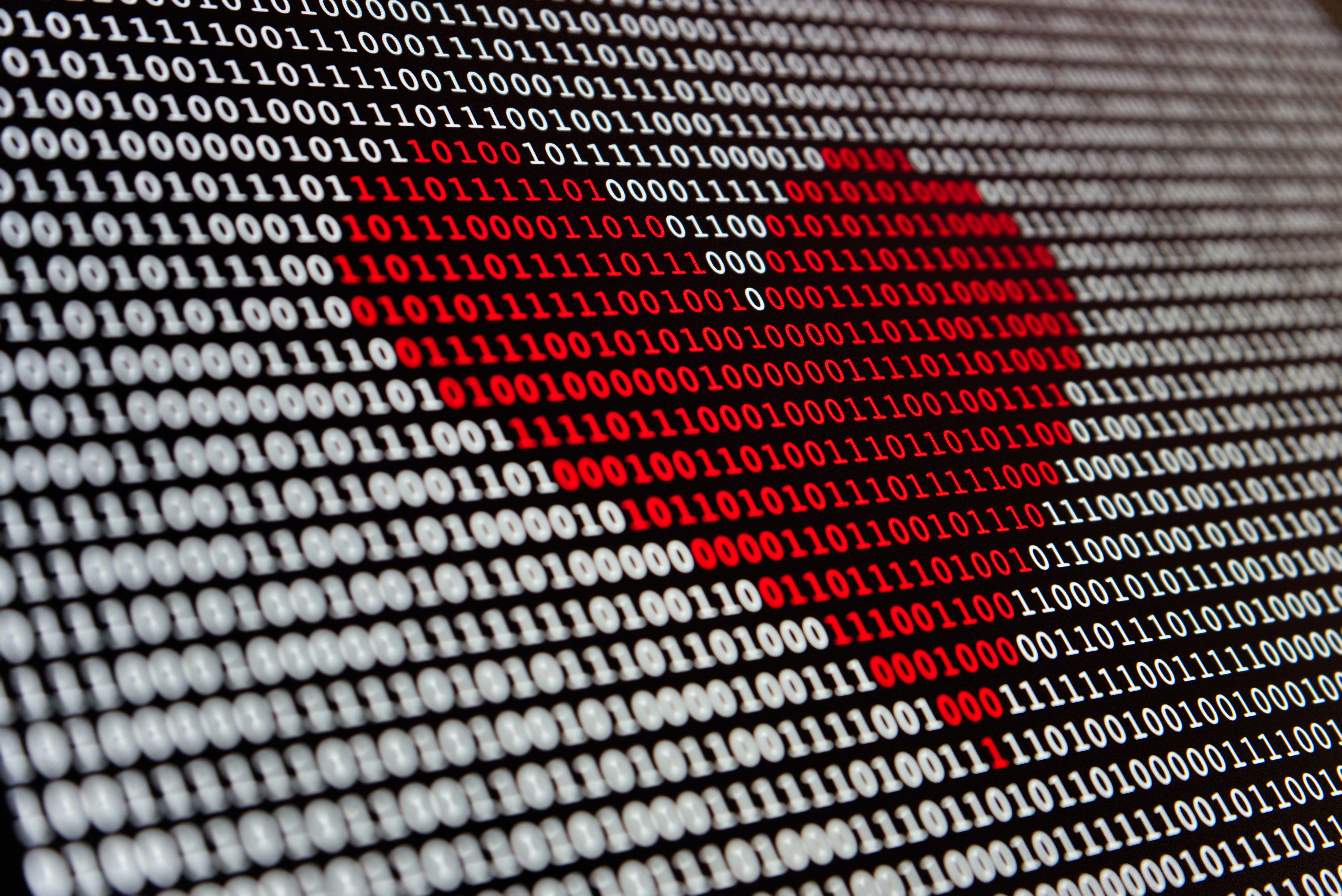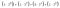# Binary — The Way Computers Read NumbersPhoto by Alexander Sinn on Unsplash

Binary (/ˈbʌɪnəri/) usually refers to something that involves two things. Many people can look at binary code and instantly associate it with computers without a second thought, but what actually is binary?

# What is Binary?

Binary is a counting system in Base 2. The counting system we use in everyday maths is in base 10. We represent our numbers as a collection of digits between 0–9 (111, 932, 53, 9, 10293, etc.). In binary, numbers are a collection of digits that are 0 and 1 (0100, 1111,1100).

Computers “read” all (or almost all) instructions in binary. Electricity flows through many transistors which can turn a switch on, or have no electricity run through the transistor leaving a switch off. This on/off structure is responsible for the binary nature of modern day computing.

# Counting Systems (Number bases)

Number bases (or as I like to call them, counting systems) start at 0 and counts up to the number N-1. For example, in our system of base 10, we count from 0,1,2,3,… all the way up to 9 which is 10 -1. After we reach the limit, we “carry a digit” over. In our case, after 9, we go to 10. The 9 resets to 0 and we carry 1 over.

If N-1 becomes larger than 9, we substitute the digits with letters. For example in base 16, the count goes 0,1,2,3,4,5,6,7,8,9,A,B,C,D,E,F. A number in base 16 would be 10AF (which would be 4271 in base 10!).

Let’s suppose we had a number 7091. This can be written as:7,091 Written in expanded form.

This is special because it means that in base 10, no other combination of numbers can have the same value of 7091. This fact is true for all numbers in any base as long as the two numbers you’re comparing are part of the same base.

Using the logic from above, we can say that Binary (or base 2) is a counting system that starts at 0 and goes up to 1. A sample number would be 1101. This can also be written as:1101 in expanded form

There is no other number that you can write in base 2 that will give you the same value as 1101.

In short, binary is one way of representing a unique number. Just as we know 620 is unique in base 10, a number like 1010 is a representation of a unique number in base 2.

# So… How do we turn our numbers into binary (and vice versa)?

Converting a binary number to a decimal number (base 10) is simple. We even did it earlier on! Let’s use the same number 1101 from earlier. We know that we can represent 1101 as:1101 in expanded form

Which can be written as 1×8 + 1×4 +0×2 + 1×1. The final thing we need to do is to add it all up together. (8+4+0+1) = 13.

Therefore our final answer is 1101 = 13 in base 10.

So… how do we do the opposite of what we did to get a binary number?

1. Find the largest power of two that’s smaller than the number
2. Subtract the power of two from the number
3. Rinse and repeat with the remainder until you reach 0

Let’s do this with the number 175. The largest power of 2 that goes into 175 is 128. To test this, we would go through from the bottom. Does 2⁴ go into 175? Yes. Does 2⁵(32) go into 175? Yes… Does 2⁷(128) go into 175? Yes. Does 2⁸(256) go into 175? No. So the largest is 2⁷.

Our next step is to do 175–128 = 47.

Now we repeat the steps

• There is no 2⁶ term
• 47–32 = 15
• There is no 2⁴ term
• 15–8 = 7
• 7–4 = 3
• 3–2 = 1
• 1–1 = 0

So we can say that 175 = 1(2⁷) + 0(2⁶) + 1(2⁵)+ 0(2⁴) + 1(2³) + 1(2²)+1(2¹) + 1(2⁰), which can be written as 10101111.

And that’s about it! Computers use these numbers in all sorts of ways: Representing characters, adding numbers, executing commands, etc. Hopefully you were able to understand what binary is and how it works. Binary is another segment of maths that often gets overlooked as “computer language” and misunderstood by many people.

# Contact me if you have any other questions!📩

Hi! I’m an 18 year old student who’s a huge computer science, programming, maths and music geek!

If you enjoyed this article, feel free to give me a follow. I plan on writing more articles to explain harder concepts in a simple fashion.

🎈Github

🎈LinkedIn

18-Year old Music, Programming and Computer Science enthusiast

## More from Jaden Shek

18-Year old Music, Programming and Computer Science enthusiast